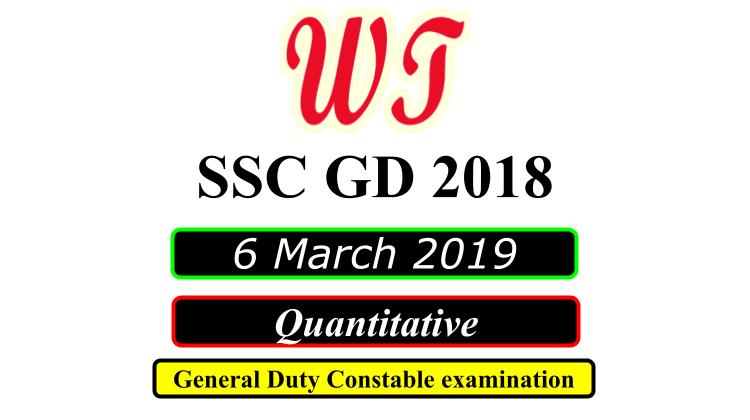Type Here to Get Search Results !

Download SSC (Staff Selection Commission) GD (General Duty), 6 March 2019 ( Math )Quantitative Questions PDF With Answers.

Table of Content

Table of Content (toc)SSC GD 6 March 2019 Quantitative Questions PDF Download Free

### SSC GD 6 March 2019 Shift 1 Math Questions

Q6:-Sixty percent of the rats included in a particular experiment were female rats. If some of the rats died during the experiment and 30% of rats died were male, what was the ratio of the death rate among the male rats to the death rate among the female rats?
A.    3 : 4
B.    9 : 14
C.    9 : 16
D.    6 : 7
Q8:-What is the fourth proportional to 3, 7 and 15?
A.    30
B.    45
C.    35
D.    25
Q9:-A sum of ₹ 5000 is invested for two years under compound interest at 10% p.a, interest being compounded annually. The interest earned (in ₹) is:
A.    2100
B.    1000
C.    1050
D.    500
Q10:-A train passes the platform in 18 seconds and a man standing on the platform in 8 seconds. If the length of the train is 100 metres, the length of the platform is:
A.    125 m
B.    140 m
C.    150 m
D.    130 m
Q11:-John travels (2/3)rd of a distance by bike and remaining 6 km on foot. How many km does he travels in the whole journey?
A.    28km
B.    18km
C.    26km
D.    14km

### SSC GD 6 March 2019 Shift 2 Math Questions

Q2:-Rohit and Dinesh are 64 km apart. Rohit can walk at a speed of 15 km/hr and Dinesh at the speed of 17 km/hr. In how many hours will they meet if they are travelling towards each other?
A.    2.5 hour
B.    2 hour
C.    3 hour
D.    1.5 hour
Q3:-A frog was, at the bottom of a 80 m deep well. It attempted to come out of it by jumping. In each jump it covered 1.15 m but slipped down by 0.75 m. The number of jumps after which it would out of the well is:
A.    200
B.    198
C.    201
D.    199
Q4:-Yashwant buys candies at 15 for ₹40 and sells them at 30 for ₹50. What is his gain or loss percent?
A.    37.5% Profit
B.    40 % Loss
C.    36 % Profit
D.    37.5% Loss
Q7:-The average age of Indian cricket team playing in the Capetown test match is 28 years. If the average age of 10 player besides the caption is 27.8 years, then the age of the Caption is:
A.    31 Years
B.    26 Years
C.    25 Years
D.    30 Years
Q8:-The third proportional to 9 and 15 is:
A.    18
B.    36
C.    27
D.    25

### SSC GD 6 March 2019 Shift 3 Math Questions

Instructions
For the following questions answer them individually
Q1:-The average age of 3 persons is 30 years. If their ages are in the ratio of 3:5:7 respectively, then the age of the eldest person is:
A.    25 Years
B.    42 Years
C.    28 Years
D.    21 Years
Q2:-The L.C.M. of two different numbers are 30. Which of the following cannot be their H.C.F.?
A.    12
B.    15
C.    6
D.    10
Q4:-On a certain sum, rate of interest per annum for the first two years is 4%. The rate of interest for next four years is 6% and for the next three years is 8%. If total simple interest earned at the end of 9 years is ₹ 1120, then the sum is:
A.    ₹2240
B.    ₹1600
C.    ₹1800
D.    ₹2000
Q7:-In a colony 5 families have 1 child, 7 families have 2 children, 8 families have 3 children and 3 families have 4 children. What is the mode of the number of children.
A.    4
B.    8
C.    5
D.    3
Q12:-The income of A and are in the ratio 5: 3. The expenses of A, B and C are in the ratio of 8: 5: 2. If C spends 2000 and B saves ₹ 700, then A saves:
A.    ₹1000
B.    ₹1500
C.    ₹500
D.    ₹250

SSC GD 2018

SSC GD Previous Year Paper

SSC GD Previous Year English Questions

SSC GD Previous Year Reasoning Questions

SSC GD Previous Year Quantitative Questions